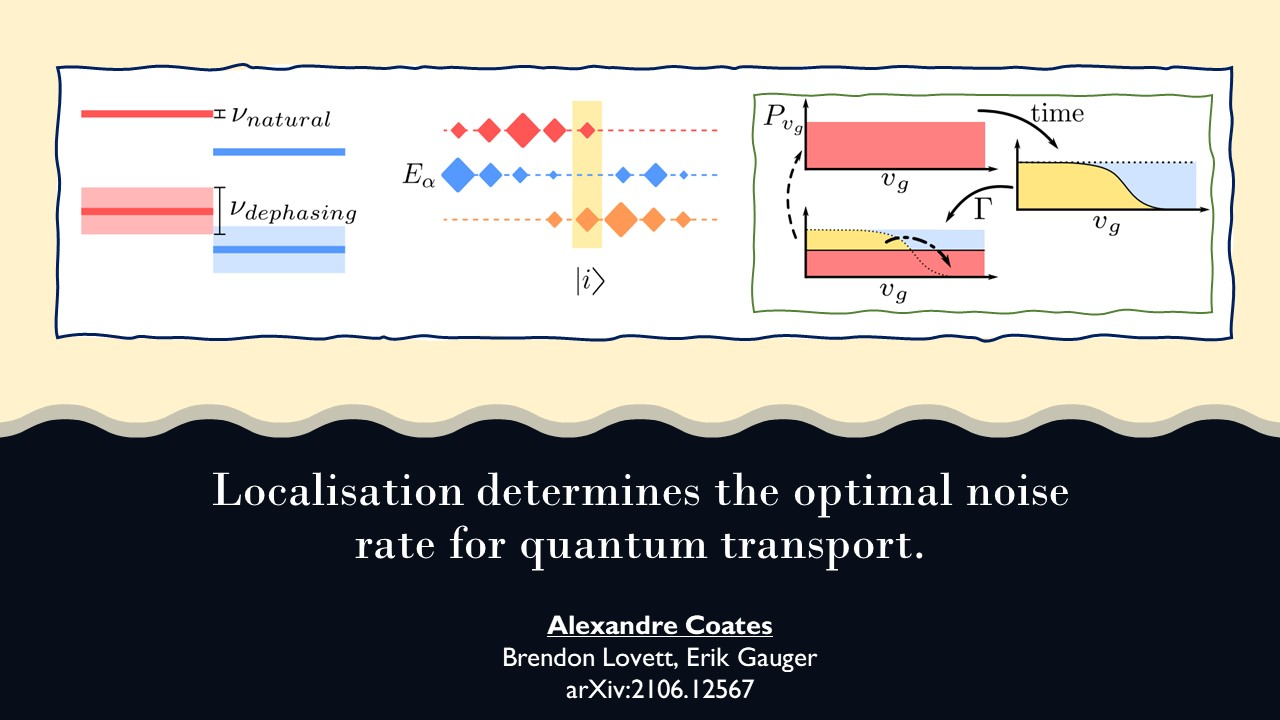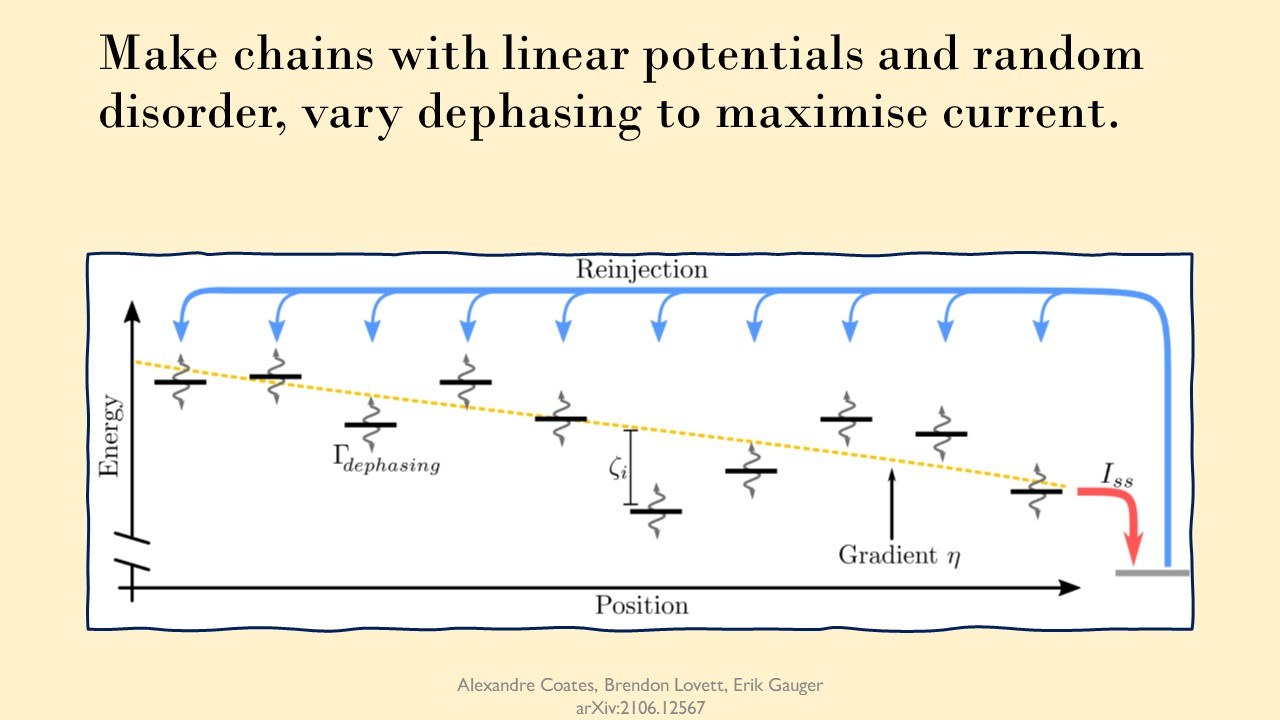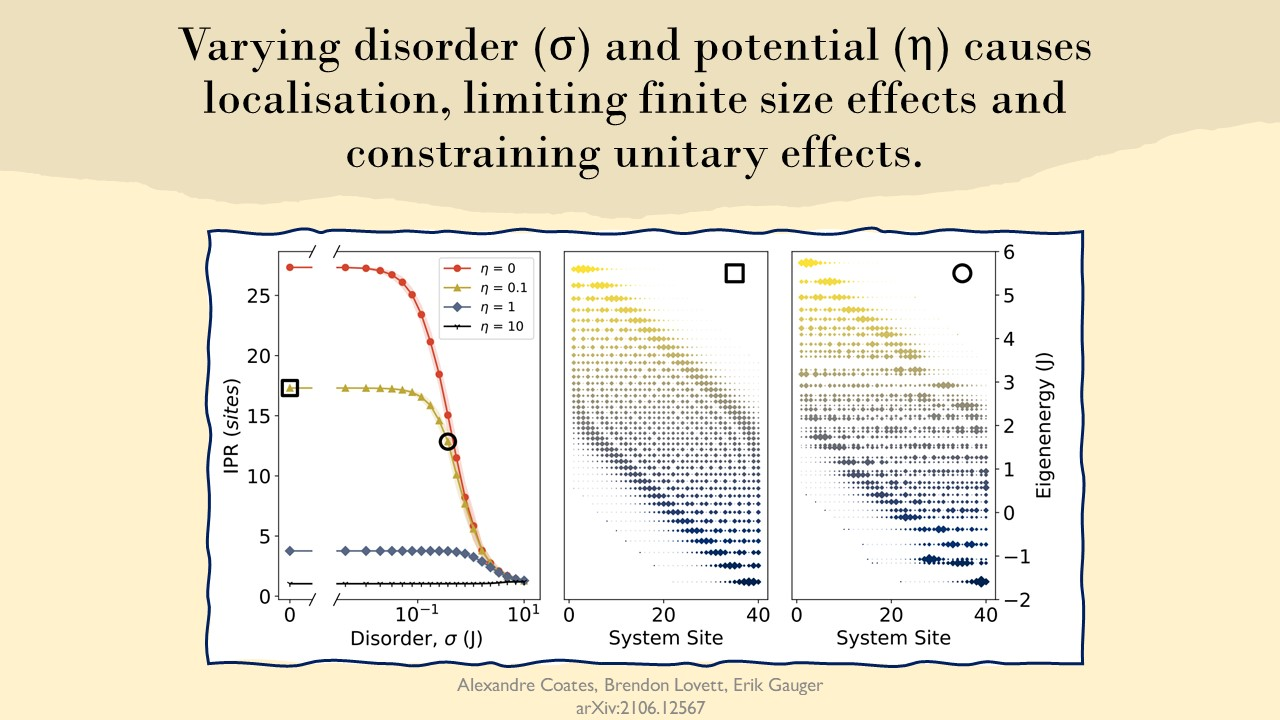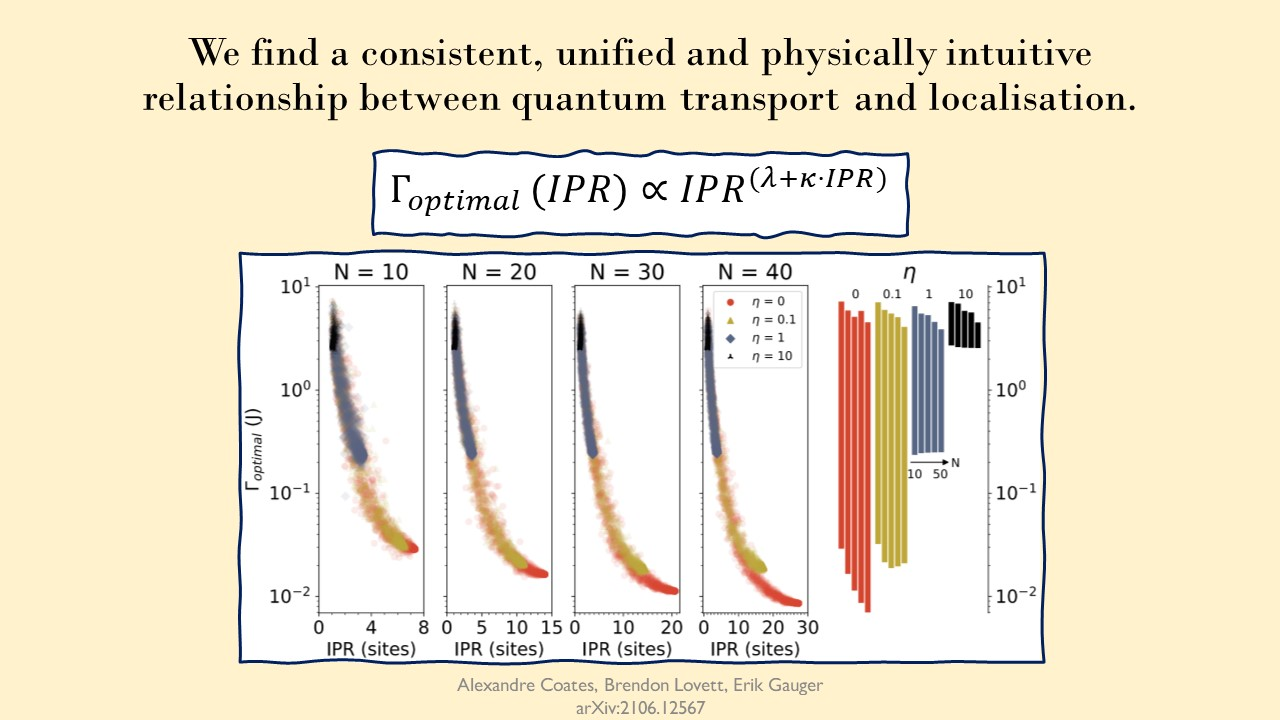## Introduction

While I’m waiting to hear back from referees on my first manuscript on localisation and Environment-Assisted Quantum Transport (ENAQT) - (you can read the preprint here). I have the opportunity now to present some of my work at QUAMP 2021 as a digital poster made of 4 slides. Along with some additional detail.

So I thought it would be good to show that here as well.

# Digital Poster - Localisation and ENAQT

## Slide 1 - relevant ENAQT mechanismsHere I show 3 relevant ENAQT mechanisms for the paper. They are linewidth broadening, the exclusive subspace, and momentum rejuvenation respectively. The first two are size-independent, being dependent only on a system’s energy differences and the linearity of the eigenbasis (meaning there is always at least 1 eigenstate with 0 presence on a given site).

Meanwhile, momentum rejuvenation is a finite size effect, dependent on the effective size of the system. Which is possible to adjust. Making it a useful probe as we can turn it from present to absent with the right adjustments. For completion’s sake I will note that momentum rejuvenation makes the following claim: a larger system wants less noise for efficient transport. There is a minimum time it takes for fast moving components of any excitation to leave the system $t_{min}$, that time scales with size $N$, and interrupting the system at a rate more frequently than $\frac{1}{t_{min}}$ is inefficient.

## Slide 2 - the set upThis investigation was carried out by modelling thousands of nearest neighbour chains. We generated them with a range of gradients $\eta$, and a range of random on-site disorders $\zeta$, sampled from Normal distributions. By varying the gradients and the width of the Gaussian distributions $\sigma$ we were able to localise the chains in various ways.

The pumping scheme was to pump onto all sites equally (the steady state equivalent of a mixed initial state), and to extract our excitons from one end of the chain. By measuring the steady state current extracted, we could then find the optimal amount of dephasing $\Gamma_{optimal}$ for each chain.

## Slide 3 - effects of localisationThe point here is very directly that, the more localised a system is, the less spread out it’s eigenstates are. In a very direct sense, that is the definition of localisation. To ensure our intuition is general we consider combinations of two localisation methods: one from applying a linear potential (Wannier-Stark) and another from applying random disorders (Anderson), both affect the eigenbasis differently.

The direct implication of localisation being that ENAQT this is that we constrain all of our ENAQT effects. Broadening linewidths does not enable many new transitions, and even more eigenstates will have no presence on any chosen site. From this we get the general tendency that prior research has described: more noise is needed to overcome the effects of more localisation.

The effects are the same, just needing a bit more effort for the same effects as in ordered systems. So we would expect a broad proportionality between the peak noise and the IPR (how many sites the average eigenstate is distributed across), which becomes the power law $\Gamma_{optimal} \propto IPR^\lambda$.

However momentum rejuvenation is a finite size effect, implying that it will disappear in disordered systems. Implying that we need a correction to the above expression.

You can play with interactive sliders below to see how the two kinds of localisation affect the eigenbasis of an $N = 10$ chain. As you can see, when the system is relatively ordered, edge effects become visible, but these disappear as localisation comes to the fore.

## Slide 4 - relating localisation and peak noise to a power lawFinally we look at chains with different lengths and see how the optimal dephasing rates change across them. We see a very consistent shape that fits very well to our amended curved power law $\Gamma_{optimal} \propto IPR^{\lambda + \kappa \cdot IPR}$, where $\lambda$ corresponds to the size independent effects, and $\kappa$ relates to the size-dependent effects, modelling the influence of momentum rejuvenation.

The far right panel shows the $\Gamma_{opt}$ range for each gradient, on each length of chain considered. Note that the gradient free ($\eta = 0$) bars show a decreasing lower range, as we expect from momentum rejuvenation. Larger systems, lower peak noise rates. However for systems with large gradients ($\eta = 1,10$) the lower limit is relatively constant across lengths, showing size dependence(and thus momentum rejuvenation) is no longer in effect.

So we show that for 1D systems that the optimal noise rate for ENAQT is dependent on the typical eigenstate width (IPR) and can be expressed simply as a power law with both size-independent and size-dependent components. Providing a cohesive, physically intuitive view of how ENAQT functions. For more details, including work applying this to chains at finite temperatures with the nonsecular Bloch Redfield Master equation, please see the preprint.

Tags:

Categories:

Updated: